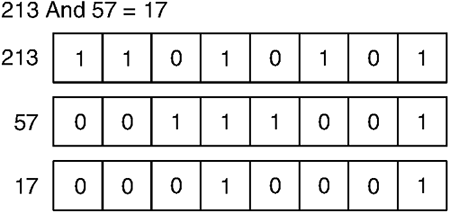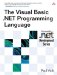# Logical and Bitwise Operators

 <  Day Day Up  >

When the operators Not , And , Or , and Xor are applied to Boolean values, they function as logical operators . That is, they perform the specified logical operation (NOT, AND, OR, or Exclusive OR) on the Boolean values. For example, the result of True Or False is True , and the result of True Xor True is False .

There are two additional logical operators: AndAlso and OrElse . AndAlso and OrElse are equivalent to And and Or , respectively, except that they can short-circuit their evaluation. This means that if the result of the operation can be determined only by evaluating the first operand, the second operand will not be evaluated. In the case of AndAlso , if the first operand evaluates to False , the second operand is not evaluated. In the case of OrElse , if the first operand evaluates to True , the second operand is not evaluated.

Short-circuiting is useful for two reasons. First, it can be used to produce more efficient code ”if the second part of a logical operation is expensive to evaluate, not evaluating it can save execution time. Also, short-circuiting can be used to simplify statements, yet avoid runtime errors. For example, compare the two ways of calculating the result in the following code.

` If x <> 0 Then   If y \ x = 10 Then     ...   End If End If If x <> 0 AndAlso y \ x = 10 Then   ... End If `

In the first case, two If statements are required because if x = 0 , y \ x will throw a System.DivideByZeroException exception. In the second case, if x = 0 , the second expression will not be evaluated and no error will occur.

When applied to the integer values, the operators Not , And , Or , and Xor function as bitwise operators , operating on the binary representation of the values. The bitwise operators execute the specific logical operation on each bit of the operand(s) to produce the result value: Not results in True if the bit is False , And results in True if both bits are True (see Figure 5-1), Or results in True if either bit is True , and Xor results in True if either bit is True , but not both.

##### Figure 5-1. And OperationThe following are some examples of the result of the bitwise operators.

` Dim a, b, c, d As Integer a = Not 143      ' Result = -144 b = 312 And 43   ' Result = 40 c = 5823 Or 412  ' Result = 6079 d = 394 Xor 123  ' Result = 497 `
 <  Day Day Up  >The Visual Basic .NET Programming Language
ISBN: 0321169514
EAN: 2147483647
Year: 2004
Pages: 173
Authors: Paul Vick

Similar book on Amazon